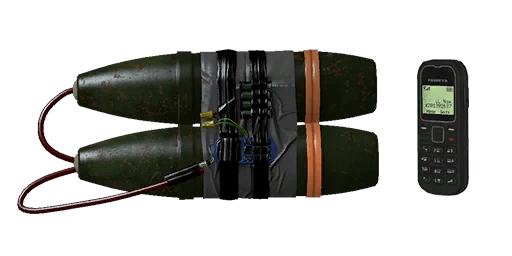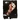An Wimprovised explosive device (IED) is usually a crudely homemade explosive device designed to be planted in roadside ambushes or used as explosive devices. Depending on the design, IEDs may be detonated remotely using a modified cell phone.

## Insurgency

{{#infoboxbuilder: | 0:Theme = INS default | 0:CustomModule = | 0:MainImageCaption = | 0:ToggleContentLongerThan = 1500

| 1:Type = Title

```| 1:Value = IED
```

| 2:Type = MainImage

```| 2:Value =```

| 3:Value =

General information

| 4:Type = Line

```| 4:Label = Inventory slot
| 4:Value = Explosive
```

| 5:Type = Line

```| 5:Label = Weapon class
| 5:Value = Remote explosive
```

| 7:Type = Line

```| 7:Label = Operators
| 7:Value =Insurgent Forces
```

| 9:Type = Line

```| 9:Label = Console ?
| 9:Value = `weapon_c4_ied`
```

| 10:Value =

Statistics
Damage

| 11:Type = Line

```| 11:Label = Damage
| 11:Value = 380
```

| 12:Type = Line

```| 12:Label = Explosion radius
```

| 12:Value = 1200

| 14:Type = Line

```| 14:Label = Max range ?
| 14:Value =
```

| 15:Type = Line

```| 15:Label = Rate of fire
| 15:Value =
```

| 16:Type = Line

```| 16:Label = Fuse time
| 16:Value = 0.5 s
```

| 17:Type = Line

```| 17:Label = Cookable?
| 17:Value =
```

| 18:Type = Line

```| 18:Label = Effect duration
| 18:Value =
```

| 19:Value =

Ammunition

| 22:Type = Line

```| 22:Label = Starting ammo
| 22:Value = 1
```

| 23:Type = Line

```| 23:Label = Reload time
| 23:Value =
```

| 29:Value =

General statistics

| 30:Type = Line

```| 30:Label = Suppression
| 30:Value =
```

| 31:Type = Line

```| 31:Label = Penetration
| 31:Value =
```

| 32:Type = Line

```| 32:Label = Optic magnification
| 32:Value =
```

| 33:Type = Line

```| 33:Label = Supply points ?
| 33:Value = 4```

| 34:Type = Line

```| 34:Label = Weight points ?
| 34:Value = 64/640
```

| 35:Type = Line

```| 35:Label = Weight
| 35:Value = 1.98 kg
```

| 36:Type = Line

```| 36:Label = Length ?
| 36:Value = 21
```

}}

The IED is an explosive featured in Insurgency.

## Insurgency: Sandstorm

{{#infoboxbuilder: | 0:Theme = IS default | 0:CustomModule = | 0:MainImageCaption = | 0:ToggleContentLongerThan = 1500

| 1:Type = Title

```| 1:Value = IED
```

| 2:Type = MainImage

```| 2:Value = File:IS IED.png
```

| 3:Value =

General information

| 4:Type = Line

```| 4:Label = Inventory slot
| 4:Value = Explosive
```

| 5:Type = Line

```| 5:Label = Weapon class
| 5:Value = Remote explosive
```

| 7:Type = Line

```| 7:Label = Operators
| 7:Value =Insurgent Forces
```

| 9:Type = Line

```| 9:Label = Console ?
| 9:Value =
```

| 10:Value =

Statistics
Damage

| 11:Type = Line

```| 11:Label = Damage
| 11:Value =
```

| 12:Type = Line

```| 12:Label = Explosion radius
| 12:Value =
```

| 14:Type = Line

```| 14:Label = Max range ?
| 14:Value =
```

| 15:Type = Line

```| 15:Label = Rate of fire
| 15:Value =
```

| 16:Type = Line

```| 16:Label = Fuse time
| 16:Value =
```

| 17:Type = Line

```| 17:Label = Cookable?
| 17:Value =
```

| 18:Type = Line

```| 18:Label = Effect duration
| 18:Value =
```

| 19:Value =

Ammunition

| 22:Type = Line

```| 22:Label = Starting ammo
| 22:Value = 1 explosive2 (LC)3 (HC)
```

| 23:Type = Line

```| 23:Label = Reload time
| 23:Value =
```

| 29:Value =

General statistics

| 30:Type = Line

```| 30:Label = Suppression
| 30:Value =
```

| 31:Type = Line

```| 31:Label = Penetration
| 31:Value =
```

| 32:Type = Line

```| 32:Label = Optic magnification
| 32:Value =
```

| 33:Type = Line

```| 33:Label = Supply points ?
| 33:Value = 3
```

| 34:Type = Line

```| 34:Label = Weight points ?
| 34:Value =
```

| 35:Type = Line

```| 35:Label = Weight
| 35:Value = 5%
```

| 36:Type = Line

```| 36:Label = Length ?
| 36:Value =
```

}}

"Remotely detonated Improvised explosive device. Throw with or plant on a surface with [key], and detonate with [key]."
— In-game description

The IED is an explosive featured in Insurgency: Sandstorm.

## Trivia

• The cell phone detonator's brand is called "Fawkya", most likely a parody of the Finnish Nokia cell phone brand.
• The name is also possibly meant as a humorous easter egg, as it bears similarity with the phrase, "fuck you".